# OOF: Finite Element Analysis of Microstructures

isotropicNext: empty Up: Element Types and their Previous: Element Types and their   Contents

Subsections

## isotropic

An isotropic element has the same stiffness independent of the loading direction or the plane on which the load is applied.

According to Figure 4.2, there are two independent elastic constants. OOF uses the Young's modulus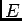and the Poisson's ratio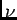for the two input variables. In the case of plane stress, the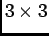stiffness matrix is: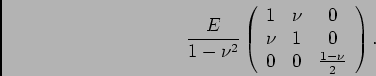For plane strain, the stiffness matrix is: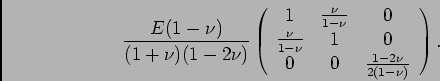### Parameters

young
The Young's modulus[stress].

poisson
The Poisson's ratio[dimensionless].

alpha
The coefficient of thermal expansion [inverse temperature].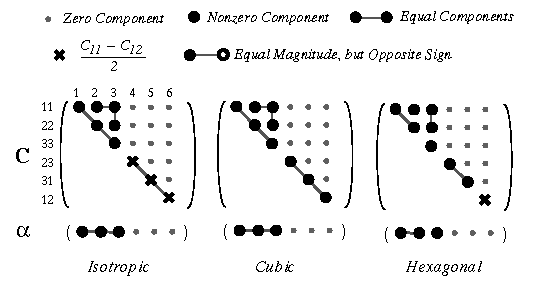/* Send mail to the OOF Team *//* Go to the OOF Home Page */